## Lyapunov Characteristic Exponent

The Lyapunov characteristic exponent [LCE] gives the rate of exponential divergence from perturbed initial conditions. To examine the behavior of an orbit around a point, perturb the system and write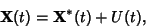(1)

where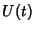is the average deviation from the unperturbed trajectory at time. In a Chaotic region, the LCE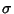is independent of. It is given by the Osedelec Theorem, which states that(2)

For an-dimensional mapping, the Lyapunov characteristic exponents are given by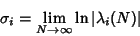(3)

for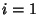, ...,, whereis the Lyapunov Characteristic Number.

One Lyapunov characteristic exponent is always 0, since there is never any divergence for a perturbed trajectory in the direction of the unperturbed trajectory. The larger the LCE, the greater the rate of exponential divergence and the wider the corresponding Separatrix of the Chaotic region. For the Standard Map, an analytic estimate of the width of the Chaotic zone by Chirikov (1979) finds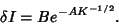(4)

Since the Lyapunov characteristic exponent increases with increasing, some relationship likely exists connecting the two. Let a trajectory (expressed as a Map) have initial conditionsand a nearby trajectory have initial conditions. The distance between trajectories at iterationis then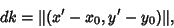(5)

and the mean exponential rate of divergence of the trajectories is defined by(6)

For an-dimensional phase space (Map), there areLyapunov characteristic exponents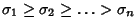. However, because the largest exponent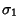will dominate, this limit is practically useful only for finding the largest exponent. Numerically, sinceincreases exponentially with, after a few steps the perturbed trajectory is no longer nearby. It is therefore necessary to renormalize frequently everysteps. Defining(7)

one can then compute(8)

Numerical computation of the second (smaller) Lyapunov exponent may be carried by considering the evolution of a 2-D surface. It will behave as(9)

socan be extracted ifis known. The process may be repeated to find smaller exponents.

For Hamiltonian Systems, the LCEs exist in additive inverse pairs, so ifis an LCE, then so is. One LCE is always 0. For a 1-D oscillator (with a 2-D phase space), the two LCEs therefore must be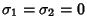, so the motion is Quasiperiodic and cannot be Chaotic. For higher order Hamiltonian Systems, there are always at least two 0 LCEs, but other LCEs may enter in plus-and-minus pairsand. If they, too, are both zero, the motion is integrable and not Chaotic. If they are Nonzero, the Positive LCEresults in an exponential separation of trajectories, which corresponds to a Chaotic region. Notice that it is not possible to have all LCEs Negative, which explains why convergence of orbits is never observed in Hamiltonian Systems.

Now consider a dissipative system. For an arbitrary-D phase space, there must always be one LCE equal to 0, since a perturbation along the path results in no divergence. The LCEs satisfy. Therefore, for a 2-D phase space of a dissipative system,. For a 3-D phase space, there are three possibilities:

1. (Integrable):,

2. (Integrable):,

3. (Chaotic):.

Chirikov, B. V. A Universal Instability of Many-Dimensional Oscillator Systems.'' Phys. Rep. 52, 264-379, 1979.